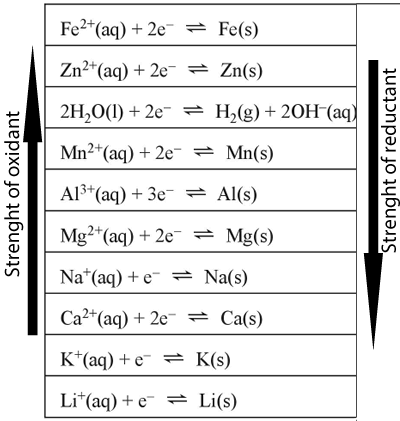Electrical energy Oxidising and reducing strengths.The diagram on the left shows the electrochemical series. This electrochemical series was derived by comparing different half cells with each other, under strictly controlled conditions, known as standard conditions. These conditions include all concentrations at 1M, at a temperature of 25°C and pressures of 1atm. We can use this series to predict what will happen if we react two half cells together. One golden rule is that "An oxidant will only react with a reductant if it is above it on the series" Such reactions are known as spontaneous reactions. For example calcium metal will react with magnesium ions but will not react with lithium ions. If more than one oxidant is present in solution, it is the strongest oxidant that will react with the strongest reductant. For example . A solution contains iron(II)nitrate, aluminium nitrate and magnesium metal. Step 1) Identify all the oxidants present Fe2+ and Al3+ and H2O Step 2) Identify all the reductants Mg Step3) Select the strongest oxidant and write the half equation as per series. The strongest oxidant is Fe2+ and the equation as per series is Fe2+(aq) + 2e <=> Fe(s) Step 4) Select the strongest reductant and reverse the equation. The strongest oxidant is Mg and the equation reversed is Mg(s)<=> Mg2+(aq) + 2e Step 5) To write the overall equation we must first make sure that the number of electrons produced during oxidation is the same as the number produced during reduction. In this example two electrons are produced and two electrons are used, the number is the same. Fe2+(aq) + 2e <=> Fe(s) Mg(s)<=> Mg2+(aq) + 2e Mg(s)+ Fe2+(aq)<=> Fe(s + Mg2+(aq) Exercises

Home